# AP Physics 2 Practice Test 15

### Test Information10 questions18 minutes

1. A battery is connected to a section of wire bent into the shape of a square. The lower end of the loop is lowered over a magnet. Which of the following orientations of the wire loop and magnet will produce a force on the wire in the positive x direction?

2.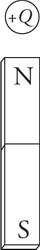A charge +Q is positioned close to a bar magnet as shown in the figure. Which way should the charge be moved to produce a magnetic force into the page on the bar magnet?

3.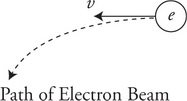In an experiment a scientist sends an electron beam through a cloud chamber and observes that the electron accelerates in a downward direction at a constant rate as shown in the figure. Which of the following could the scientist conclude?

4.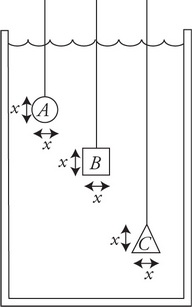A sphere, cube, and cone are each suspended stationary from strings in a large container of water as shown in the figure. Each has a width and height of x. Which of the following properly ranks the buoyancy force on the objects? (Assume the vertical distance between points A and B is small.)

5.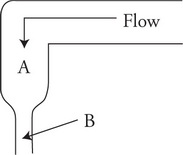Bubbles in a carbonated liquid drink dispenser flow through a tube as shown in the figure. Which of the following correctly describes the behavior of the bubbles as they move from point A to point B? The vertical distance between points A and B is small.

6. The circumference of a helium-filled balloon is measured for three different conditions: at room temperature, after being in a warm oven for 30 minutes, and after being in a freezer for 30 minutes. A student plotting the circumference cubed C3 as a function of temperature T, should expect to find which of the following?

7. A group of physics students has been asked to confirm that air exhibits properties of an ideal gas. Using a sealed cylindrical container with a movable piston, baths of cool and warm water, a thermometer, a pressure gauge, and a ruler, the students are able to produce this table of data.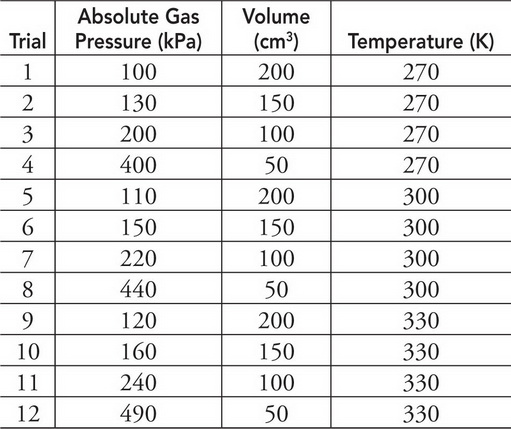Which of the following data analysis techniques, when employed by the students, could be used to verify ideal gas behavior of air?

8.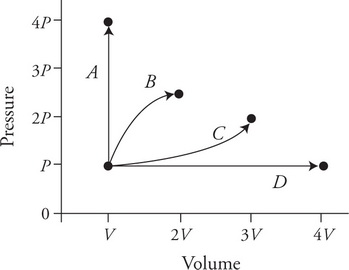A gas is initially at pressure P and volume V as shown in the graph. Along which of the labeled paths could the gas be taken to achieve the greatest increase in temperature?

9. It is observed that sounds can be heard around a corner but that light cannot be seen around a corner. What is a reasonable explanation for this observation?

10.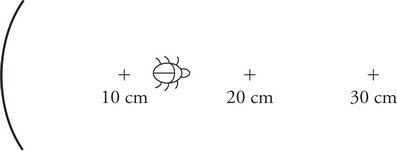A bug crawls directly away from a mirror of focal length 10 cm as shown in the figure. The bug begins at 13 cm from the mirror and ends at 20 cm. What is happening to the image of the bug?